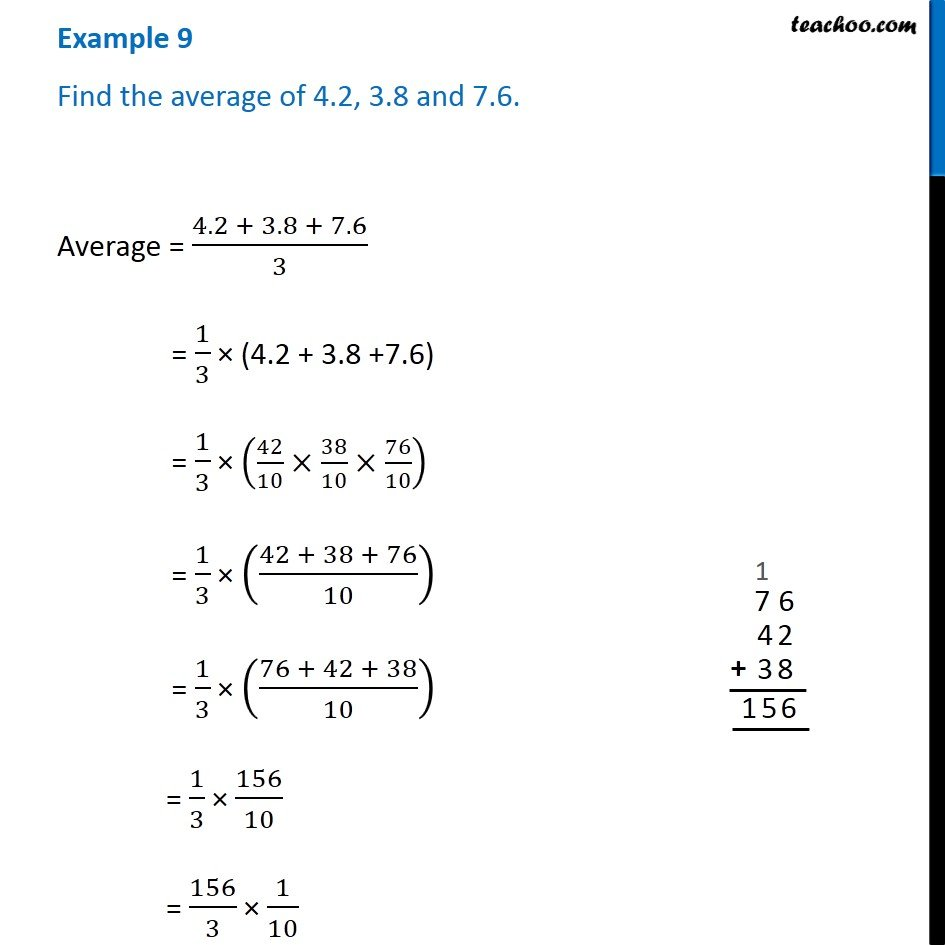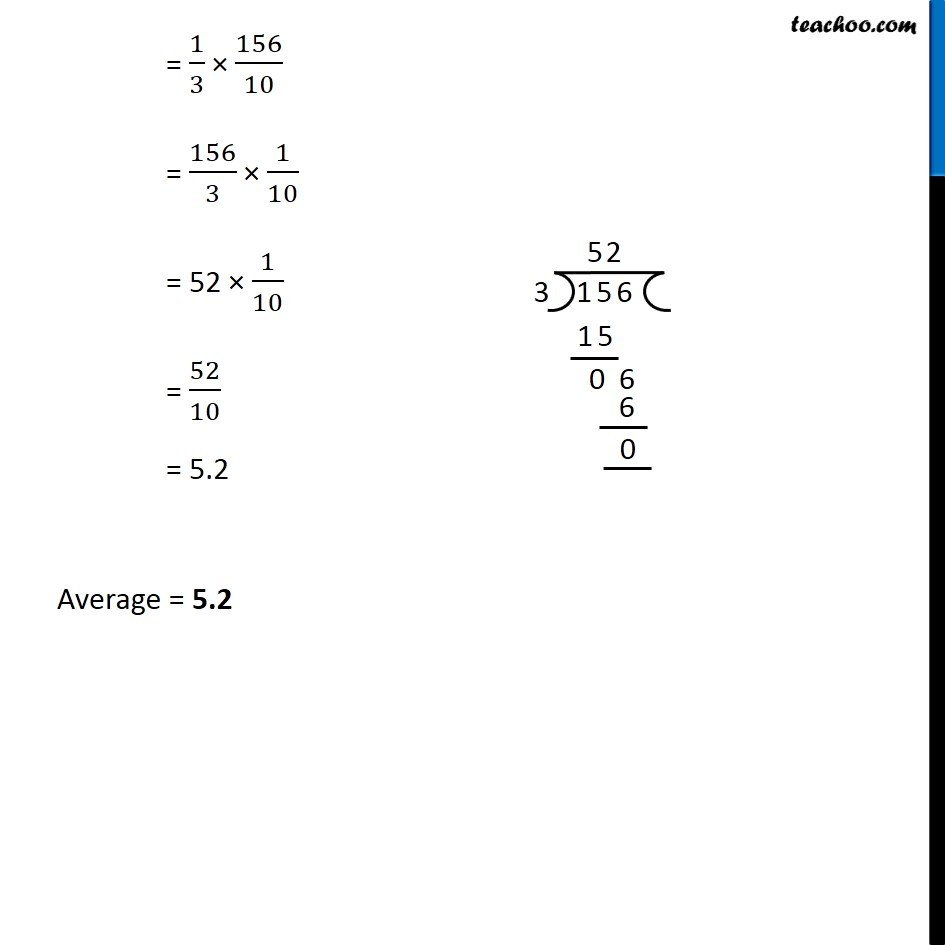Division of Decimals - Statement Questions

Chapter 2 Class 7 Fractions and Decimals
Concept wise641 students have Teachoo Black. What are you waiting for?

### Transcript

Example 9 Find the average of 4.2, 3.8 and 7.6.Average = (4.2 + 3.8 + 7.6)/3 = 1/3 (4.2 + 3.8 +7.6) = 1/3 (42/10 38/10 76/10) = 1/3 ((42 + 38 + 76)/10) = 1/3 ((76 + 42 + 38)/10) = 1/3 156/10 = 156/3 1/10 = 1/3 156/10 = 156/3 1/10 = 52 1/10 = 52/10 = 5.2 Average = 5.2